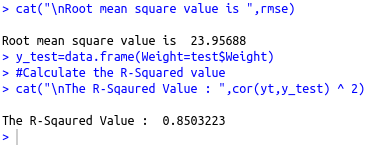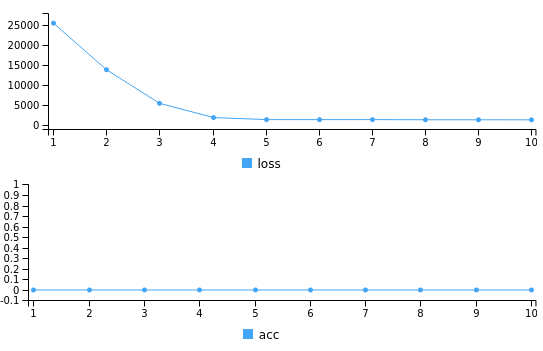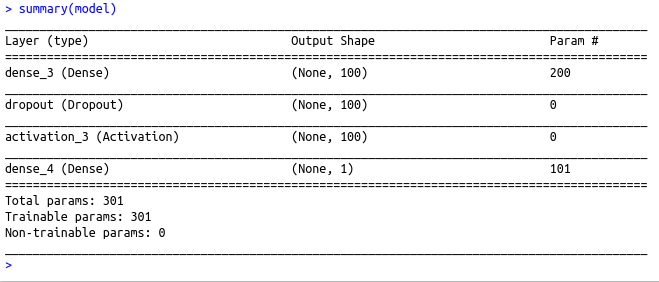• #5, First Floor, 4th Street Dr. Subbarayan Nagar Kodambakkam, Chennai-600 024 Landmark : Samiyar Madam
• pro@slogix.in
• +91- 81240 01111

#### Social List

##### How to perform Linear regression in R using Deep Neural network?
###### Description

To perform linear regression in R using deep neural network

library(keras)

#### Functions Used

model <- keras_model_sequential() - Initialize a keras sequential model layer_dense() – Add a input,output or hidden layer

layer_activation() – Activation function for the layer
compile() – To compile the model
fit() – To fit the model using the train set
predict() – To predict using the test set

###### Process

Convert the categorical variables to equivalent numeric classes

Split the data set as train set and test set

Initialize the keras sequential model

Build the model with input layers,hidden layers and output layer as per the data size along with the activation function(here 1 I/P layer with relu activation and 1 O/P layer with no activation(for linear))

Compile the model with required loss,metrics and optimizer(here loss=mean square error,optimizer=adam,metrics=accuracy)

Fit the model using the train set

Predict using the test set

Evaluate the metrics

###### Sapmle Code

library(caret)
library(keras)
#Setting seed to reproduce results of random sampling data
set.seed(100)
#Train set
train_ind<-createDataPartition(data\$Weight,p=0.8,list=FALSE) train_data<-data[train_ind,] xtrain=train_data[,2] ytrain=train_data\$Weight #Test set test=data[-train_ind,] xtest=test\$Height ytest=test\$Weight #defining a keras sequential model model <- keras_model_sequential() #Define a neural network with 1 input layer with 100 neurons and 1 output layer model %>%
layer_dense(units = 100, input_shape = 1) %>%
layer_dropout(rate=0.4)%>%
layer_activation(activation = ‘relu’) %>%
layer_dense(units = 1)

#compiling the defined model with metric = accuracy and optimiser as adam.
model %>% compile(
loss = ‘mean_squared_error’,
metrics = ‘accuracy’
)
#Summary of the model
summary(model)

#fitting the model on the training dataset
model %>% fit(xtrain, ytrain, epochs = 10, batch_size = 128)

#Predict using the Test data
yt=predict(model,xtest)

#Calculate the RMSE value
e=(ytest)-(yt)
err=e*e
rmse=sqrt(mean(err))
cat(“\nRoot mean square value is “,rmse)
y_test=data.frame(Weight=test\$Weight)
#Calculate the R-Squared value
cat(“\nThe R-Sqaured Value : “,cor(yt,y_test) ^ 2)

###### Screenshots